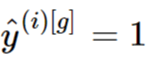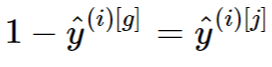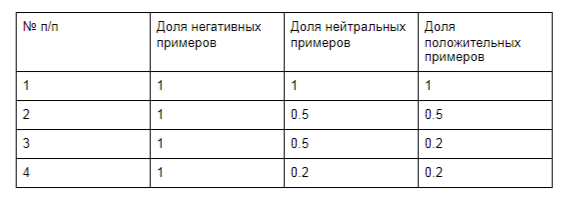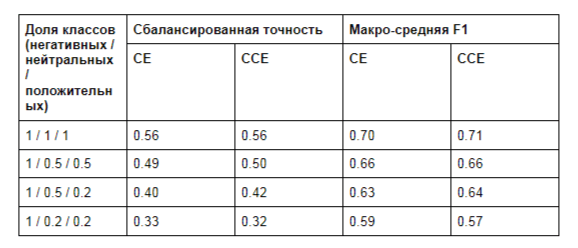Testing complementary cross-entropy in text classification problems

Earlier this year, I. Kim and co-authors published an article , which proposed a new loss function for classification problems. According to the authors, it can be used to improve the quality of models in both balanced and unbalanced classification problems in combination with standard cross-entropy.

Classification is sometimes necessary, for example, when creating recommender systems, so the described method is interesting both from an academic point of view and in the context of solving business problems.

In our article, we will check how complementary cross-entropy affects the task of classifying text on an unbalanced dataset. Our goal is not to conduct extensive experimental research or create a ready-to-use solution, but to assess its prospects. Please note that the code described in the article may not be suitable for use on the project and may require some improvement.Preliminary analysis

Before diving into the design and implementation of the experiment, let’s look at the loss function formula and analyze what we can expect from it.

In the article, the complementary cross-entropy is defined as follows:and the total loss function used in training the model is the sum with the standard cross-entropy:First of all, note that the denominators can become 0 when the model perfectly predicts the correct class and… To avoid division by zero, we add a very small ε to the denominator so that it never becomes 0.

Another problem occurs when the model is completely wrong and… In this case, the expression under the logarithm becomes 0, which makes the entire expression undefined. Here we will use the same approach, adding a small ε to avoid the zeros.

When the task includes only two classes, the expressionalways holds, and all complementary cross-entropy is always zero. So it only makes sense if the number of classes in the problem is three or more.

Finally, it is not possible to use logits directly with this loss function, since it contains a subtraction operation under the logarithm. This can potentially lead to numerical instability in the learning process.

Experiment design

Keeping all of the above in mind, you can start developing an experiment.

We will use a simple classification dataset with Kaggle … It involves the task of grading sentiment with five classes. However, extreme classes (very negative and very positive) and their milder counterparts are usually attributed to very similar texts (see Figure 1 for an example). This is probably due to the specific procedure for generating this dataset.Figure: 1. Examples of texts of the same type assigned to different classes.

To make things easier, we will reassign the classes and make three: negative, neutral, positive.

We would like to test how the loss function affects the performance of the models with several different degrees of class imbalance, so we will sample to achieve the desired class proportions. We will keep the number of negative examples constant and reduce the number of neutral and positive examples relative to him. The specific proportions used are shown in Table 1. This approach seems quite realistic, since, for example, in the field of reviews of goods or services, users are more likely to publish negative reviews than neutral and positive ones.

We would like to test how the loss function affects the performance of the models with several different degrees of class imbalance, so we will sample to achieve the desired class proportions. We will keep the number of negative examples constant and reduce the number of neutral and positive examples relative to him. The specific proportions used are shown in Table 1. This approach seems quite realistic, since, for example, in the field of reviews of goods or services, users are more likely to publish negative reviews than neutral and positive ones.Table 1. Class proportions used in experiments. Coefficients are given relative to the number of negative examples.

We will compare the complementary cross-entropy with the standard cross-entropy without class weights. We will also not consider other approaches to solving class imbalance, such as upsampling and downsampling, and the addition of synthetic and / or augmented examples. This will help us keep the experiment succinct and simple.

Finally, we split the data into train, validation and test sets in a ratio of 0.7 / 0.1 / 0.2.

We use balanced cross-entropy as the main performance metric of the model, thus following the authors of the original article. We also use macro-averaged F1 as an additional metric.

Project details

The source code of our implementation, including data preprocessing, loss function, model and experiments, is available on GitHub … Here, we’ll just look at its key points.

We are using the PyTorch framework for this experiment, but the same results can be easily reproduced using TensorFlow or other frameworks.

The implementation of the complementary cross-entropy function is pretty straightforward. We are using cross_entropy from PyTorch for standard cross entropy, so our loss function takes logits as input. Then she translates them into probabilities to calculate the complementary part.

Data preprocessing includes standard tokenization using the model ru from SpaCy.

The model we are using is a bidirectional LSTM with one fully connected layer on top of it. Dropout applies to LSTM embeddings and outputs. The model does not use pre-trained embeddings and optimizes them during training.

Details of the training process: batch size 256, learning rate 3e-4, embedding size 300, LSTM size 128, dropout level 0.1, training for 50 epochs, stopping after 5 epochs without quality improvement on validation. We use the same parameters for both complementary and standard cross-entropy experiments.

Experimental resultsTable 2. Results of experiments. CE for the experiment with standard cross-entropy and CCE for the complementary.

As can be seen from the table, the complementary cross-entropy does not give significant improvements over the standard cross-entropy function at any degree of class imbalance. A gain of 1–2 percentage points can be interpreted as random fluctuations due to the probabilistic nature of the learning process. We also do not see any improvement in the quality of the model with the new loss function compared to the standard model.

Another problem of complementary cross-entropy can be seen in the plots of the loss function (Fig. 2).Figure: 2. Loss plots for degrees of imbalance 1 / 0.2 / 0.2 (orange) and 1 / 0.5 / 0.5 (green).

As you can see, the values ​​fell far into the negative area. This is probably due to the problem of numerical instability that we discussed earlier. Interestingly, the values ​​for validation remain unchanged.

Finally

We described our experiments on the application of complementary cross-entropy to the problem of classifying texts with varying degrees of class imbalance. In experiments, this loss function did not provide any significant advantage over the standard cross-entropy in terms of the quality of the resulting models. Moreover, the loss demonstrated theoretical and experimental problems that make it difficult to use in real projects.

Notes

 Y. Kim et al, 2020. Imbalanced Image Classification with Complement Cross Entropy. arxiv.org/abs/2009.02189
 www.kaggle.com/c/sentiment-analysis-on-movie-reviews/overview
 github.com/simbirsoft/cce-loss-text